# Trigonometry Simplify – sin^4x – cos^4x

I thought I’d post this quick simplification using trig identities since it gave me a bit of a run for my money.  Partially so I remember it better and second, to lend a bit of help to those who might be stuck.

The Problem: Simplify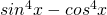Now, how hard could this be?!?  As it turns out, it’s not too bad, but the trick is in factoring what we have.

Factor things out: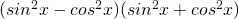Great!  Now that we have this in better form we need to bring in a couple of trig identities which will help us solve this and one that I’m going to cite because it could trick you!

Pythagorean Identity 1:Pythagorean Identity 2: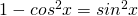Pythagorean Identity 3: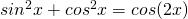(This is the tricky one!  As much as it seems like we could use this identity we would end up loosing terms.  Don’t use this)

Now, things should start looking a bit simpler to solve.  So, here we go starting with what we factored:

1)2) Use Identity 1 to substitute for the first term: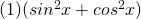3) Use Identity 2 to substitute for the first part of the second term:4) Now combine the two like terms: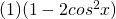5) And so we have it!  The final result is: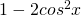Now, looking back, if you notice in identity 3, if you use it, you end up with a term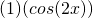which multiplied out you loose the negative and the 2 in front of cos.  This is a big problem because if you did it this way, what you get in the end does not equal what you had in the beginning and thus, isn’t a valid way to simplify this problem.  As a result, you must use identities 1 and 2 as shown in the 5 steps above.

Cheers!

### 4 Responses

1.someone October 29, 2015 / 11:26 pm

sinx^2-cosx^2=1
wrong… the identity is sinx^2+cos^2x=1

2.Jacob March 20, 2016 / 12:30 pm

I believe your first Pythagorean identity is wrong but that is coming from a freshman in college trig.

3.lydia September 20, 2016 / 6:31 pm

But sin^2x – cos^2x doesn’t equal one – – – sin^2x + cos^2x equals 1…

4.Bobbi October 4, 2016 / 2:30 pm

the Pythagorean theorem states that a^2+b^2=c^2. Using the unit circle, we can substitute to get sin^2x+cos^2x=1. Where did you get the identity “sin^2x-cos^2x=1”? Using your identity #1, we would add cos^2x to each side to get the second identity, not subtract it, so if your identity #1 is correct, then your identity #2 would be “sin^2x=1+cos^2x”. Your steps are sound, but something is up with what you have listed as the correct identities.